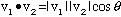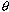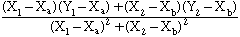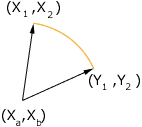Subject: 2-D Graphing

I have the following information Given.

(X1, Y1) Origional Point
(X2, Y2) Origional Point After a Rotation
(Xa, Xb) Center of Rotation

What formula would I use to figure out the angle the point was rotated?

Hi Jay,
 We suggest a vector approach to this problem. If v1 and v2 are the two vectors in the diagram thenwhereis the angle between them. Since the two vectors have the same length,is the angle whose cosine isCheers,
Chris and Harley
Go to Math Central